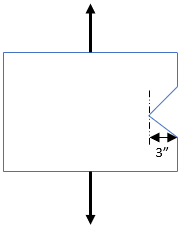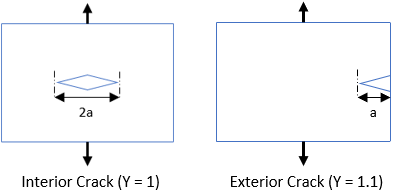## Material Crack

In the shown figure, a brittle specimen is loaded with an applied stress of 350 psi (in tension). What is the resulting stress intensity for the crack?Hint
Fracture toughness:
$$K_{IC}=Y\cdot \sigma \cdot \sqrt{\pi (a)}$$$where $$Y$$ is the geometrical factor, $$\sigma$$ is the applied engineering stress, and $$a$$ is the crack length. Hint 2Fracture toughness is the stress intensity of when a brittle material will fail due to the combination of an applied stress and crack length. $$K_{IC}=Y\cdot \sigma \cdot \sqrt{\pi (a)}$$$
where $$K_{IC}$$ is fracture toughness, $$Y$$ is the geometrical factor, $$\sigma$$ is the applied engineering stress, and $$a$$ is the crack length.Based on the problem statement:
• $$\sigma=350\:psi$$
• $$Y=1.1$$ (since an exterior crack was produced)
• $$a=3"$$
Therefore,
$$K_{IC}=1.1\cdot 350psi \cdot \sqrt{\pi (3in)}=1182\:psi\cdot in^{1/2}$$$$$1182\:psi\cdot in^{1/2}$$$